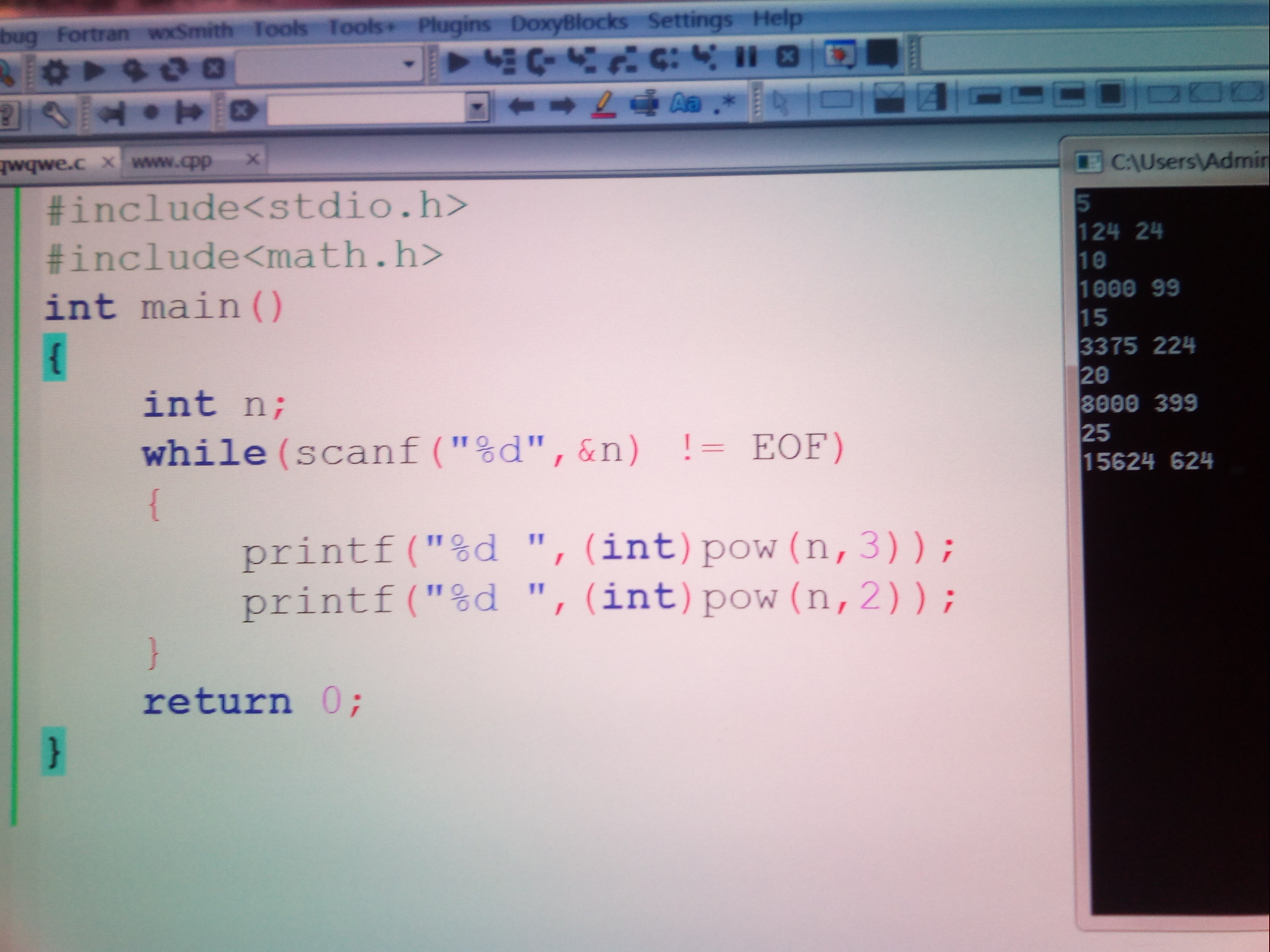``````#include<stdio.h>
#include<math.h>
int main()
{
int n;
while(scanf("%d",&n) != EOF)
{
printf("%d ",(int)pow(n,3));
printf("%d ",(int)pow(n,2));
}
return 0;
}
``````

2个回答

printf("%d ",(int)(pow(n,3) + 0.5));

``````    int n = 5;

printf("%d ",(int)pow(n,3.0));
printf("%d ",(int)pow(n,2.0));

``````